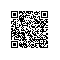# Linq使用Group By

1.简单形式：
1. var q =
2. from p in db.Products
3. group p by p.CategoryID into g
4. select g;

2.最大值

1. var q =
2. from p in db.Products
3. group p by p.CategoryID into g
4. select new {
5. g.Key,
6. MaxPrice = g.Max(p => p.UnitPrice)
7. };

3.最小值

1. var q =
2. from p in db.Products
3. group p by p.CategoryID into g
4. select new {
5. g.Key,
6. MinPrice = g.Min(p => p.UnitPrice)
7. };

4.平均值

1. var q =
2. from p in db.Products
3. group p by p.CategoryID into g
4. select new {
5. g.Key,
6. AveragePrice = g.Average(p => p.UnitPrice)
7. };

5.求和

1. var q =
2. from p in db.Products
3. group p by p.CategoryID into g
4. select new {
5. g.Key,
6. TotalPrice = g.Sum(p => p.UnitPrice)
7. };

1.计数

1. var q =
2. from p in db.Products
3. group p by p.CategoryID into g
4. select new {
5. g.Key,
6. NumProducts = g.Count()
7. };

2.带条件计数

1. var q =
2. from p in db.Products
3. group p by p.CategoryID into g
4. select new {
5. g.Key,
6. NumProducts = g.Count(p => p.Discontinued)
7. };

3.Where限制

1. var q =
2. from p in db.Products
3. group p by p.CategoryID into g
4. where g.Count() >= 10
5. select new {
6. g.Key,
7. ProductCount = g.Count()
8. };

4.多列(Multiple Columns)

1. var categories =
2. from p in db.Products
3. group p by new
4. {
5. p.CategoryID,
6. p.SupplierID
7. }
8. into g
9. select new
10. {
11. g.Key,
12. g
13. };

5.表达式(Expression)

1. var categories =
2. from p in db.Products
3. group p by new { Criterion = p.UnitPrice > 10 } into g
4. select g;

var 一般分组 = from c in ctx.Customers
group c by c.Country into g
where g.Count() > 5
orderby g.Count() descending
select new
{

};

SELECT [t1].[Country], [t1].[value3] AS [顾客数]
FROM (
SELECT COUNT(*) AS [value], COUNT(*) AS [value2], COUNT(*) AS [value3], [t0].[Country]
FROM [dbo].[Customers] AS [t0]
GROUP BY [t0].[Country]
) AS [t1]
WHERE [t1].[value] > @p0
ORDER BY [t1].[value2] DESC
-- @p0: Input Int32 (Size = 0; Prec = 0; Scale = 0) 

var 匿名类型分组 = from c in ctx.Customers
group c by new { c.City, c.Country } into g
orderby g.Key.Country, g.Key.City
select new
{

};

var 按照条件分组 = from o in ctx.Orders
group o by new { 条件 = o.Freight > 100 } into g
select new
{

};使用钉钉扫一扫加入圈子
+ 订阅